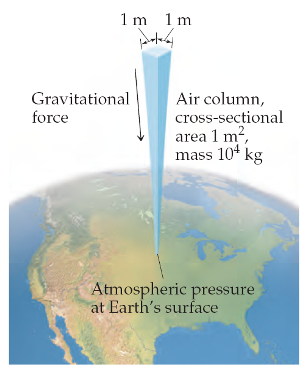# Problem: Assume the top of your head has a surface area of 25 cm 25 cm cm.Calculating atmospheric pressure.If you estimate this area to be 95 in.2, what is the force in pounds?

###### FREE Expert Solution

F = PxA###### Problem Details

Assume the top of your head has a surface area of 25 cm 25 cm cm.Calculating atmospheric pressure.

If you estimate this area to be 95 in.2, what is the force in pounds?